Updated on 20 Oct 2021

# Data Types in PythonMartin Ganchev
Published on 16 Jul 2018 8 min read

The data types in Python that we will look at in this tutorial are integers, floats, Boolean, and strings.

If you are not comfortable with using variables in Python, this article can change that. Since there are many programmers who are good at math, let’s take a look at the numeric data types in Python.

## Numeric Data Types in PythonWhen programming, if you say that a variable has a numeric value, you are being ambiguous. This is because numbers can be either integers or floats (floating points).

## Data Types: Ints

Integers are positive or negative whole numbers without a decimal point. I believe in learning through doing, so let me show you what you can do with integers. Let’s create x1 and bind to it the value of 5.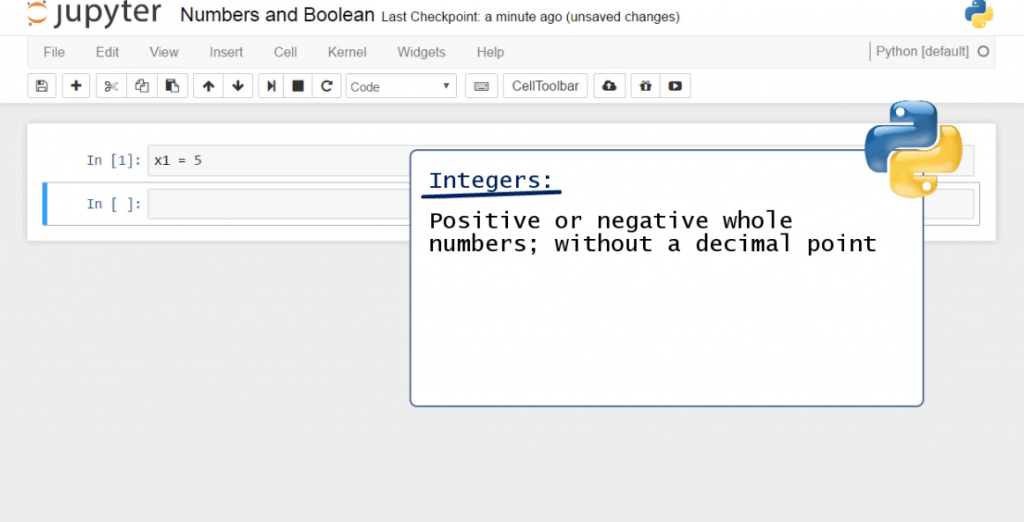Now, x1 is an integer. In fact, there is a specific function in Python that can prove this is correct.  It is called type().

### The type() Function

We can apply the type() function on different data types in Python following this rule. Within the brackets, we must place the name of the variable whose type of value we want to verify. So, in this case, we’ll type x1.

After executing the code, the result we obtain is “int”, which indicates the value is an integer.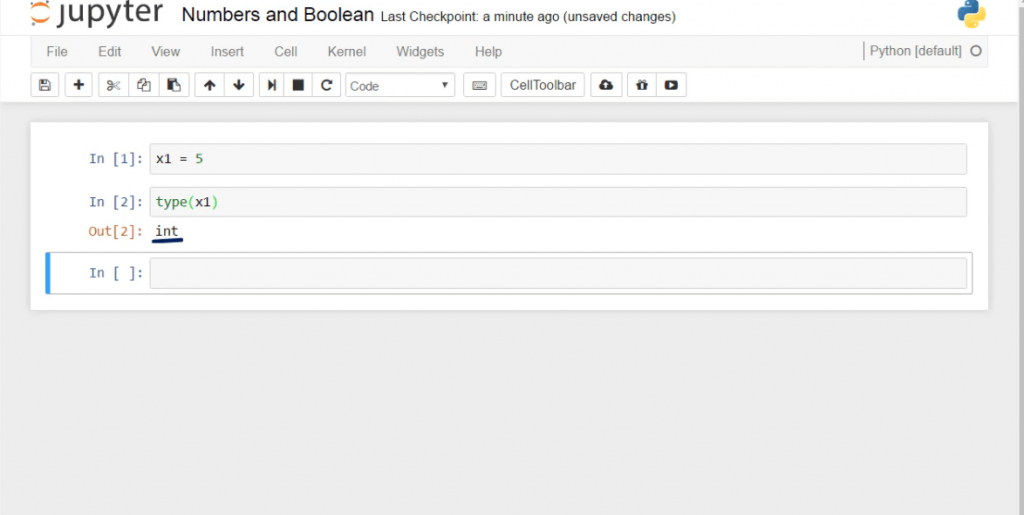The type() function can also be applied directly to a value instead of a variable. For instance, if we write type(-6), Python will correctly point out that -6 is an integer.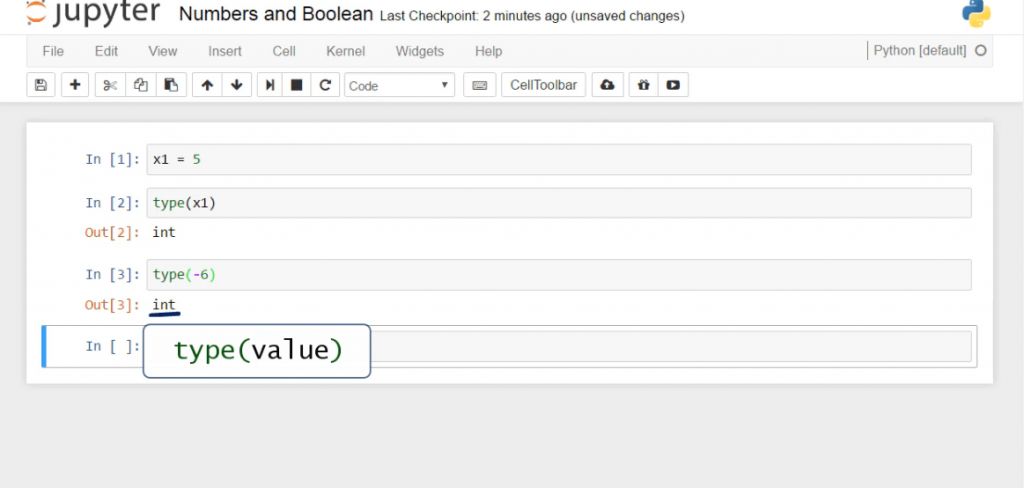## Data Types: Floats

Now, let’s assign the value of 4.75 to a new variable, x2. We can check its type by using the type() function again.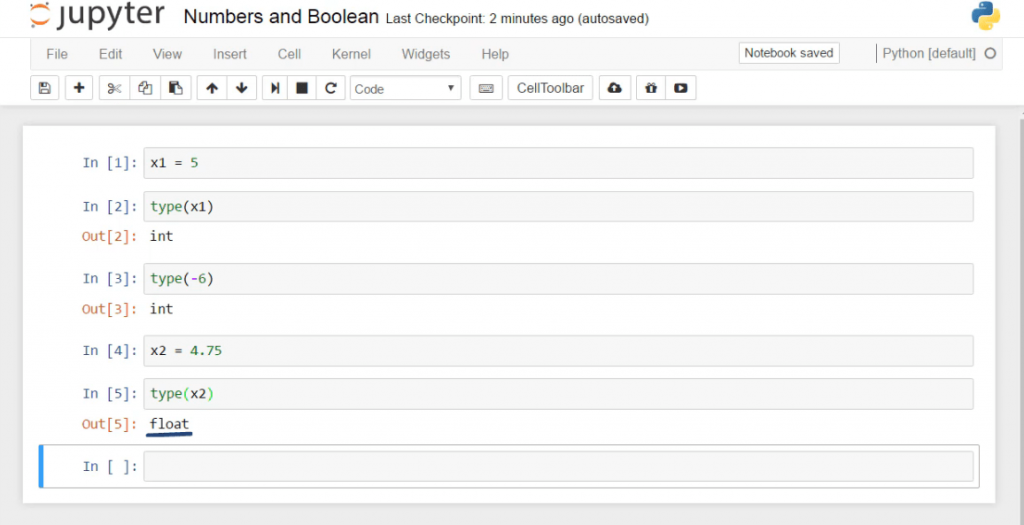This is a float.

Floating points, or as you’ll more frequently hear - floats, are real numbers. Hence, they have a decimal point. 4.75 is such a number so Python reads it as a float.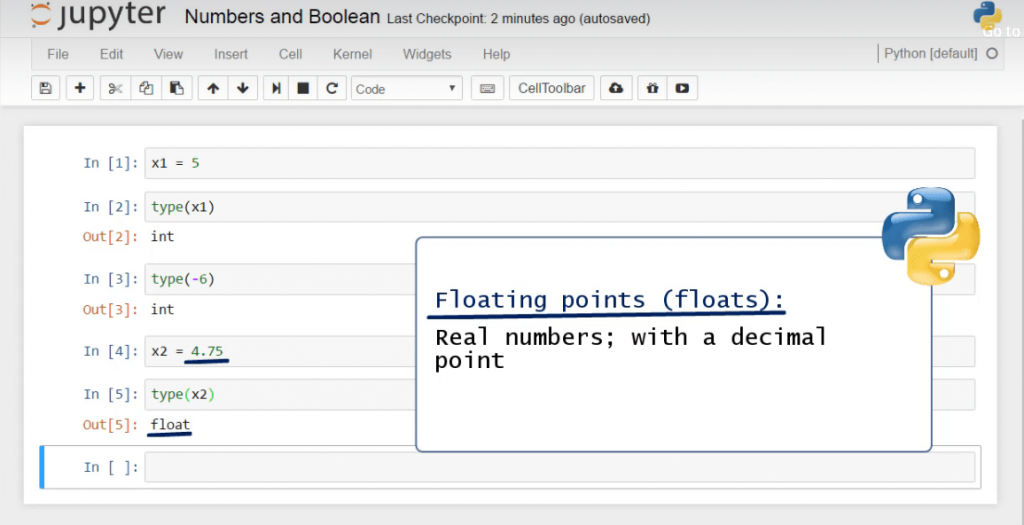Let’s look at two other built-in functions, int()and float().

### The int() Function

int() transforms the variable into an integer. That’s why 4.75 turns into 4 after executing the code shown in the picture.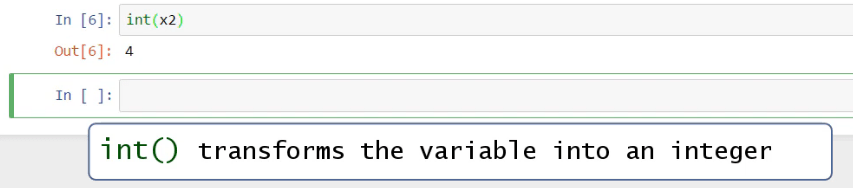### The float() Function

float(), instead, will add a decimal point to the integer or Boolean value and will turn it into a float.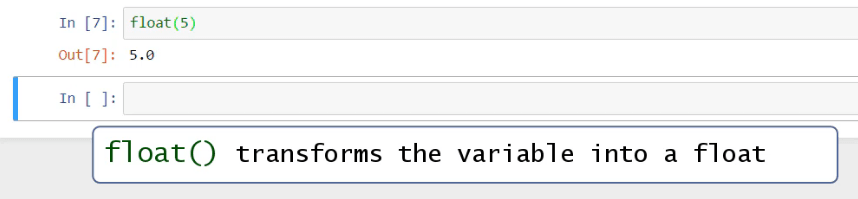## Boolean Data Type in Python

Not all variables should assume numeric values. An example of such type of values is the Boolean type. In Python, this means a True or False value, corresponding to the machine’s logic of understanding 1s and 0s, on or off, right or wrong, true or false. Let’s create a new variable, x3, and assign to it the value True. If we check its type with the type() function, we get the output ‘bool’. This means that x3 is a Boolean.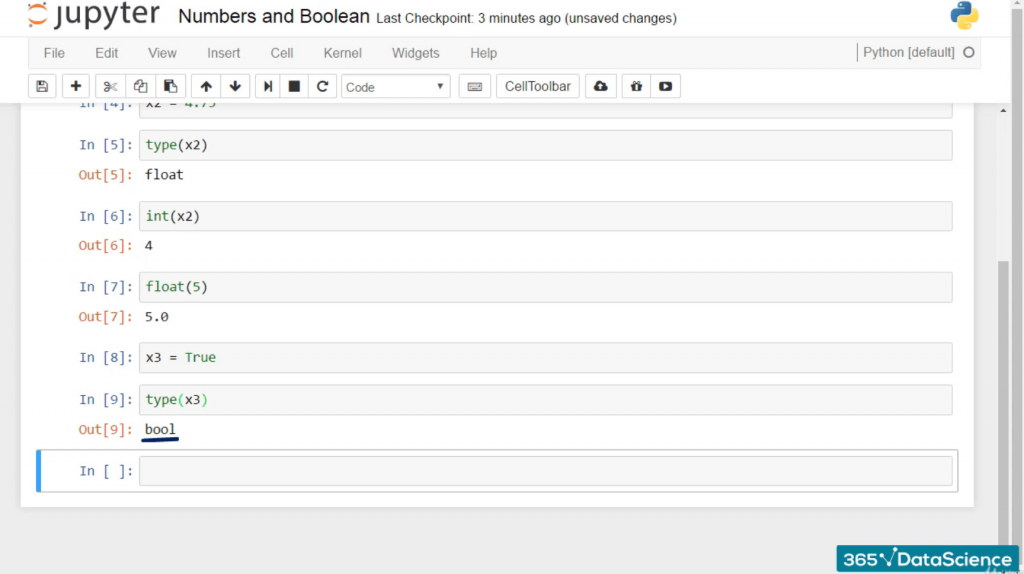Side note:  An important detail you should remember is that you have to type True or False with capital letters! Otherwise, Python won’t recognize your variable as a Boolean and will display an error message.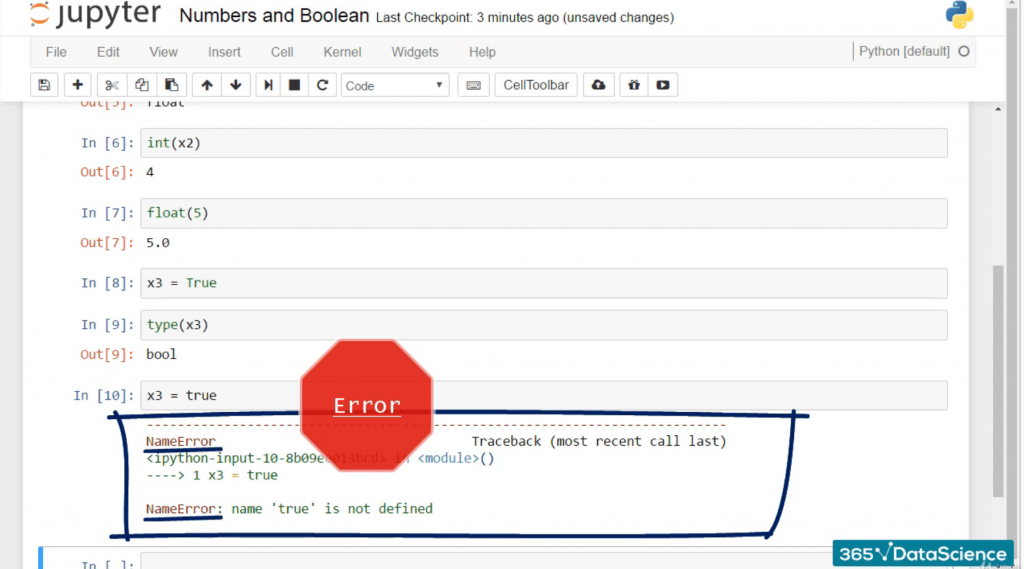So, to sum up, the two Boolean values a variable can have are True or False, and they must be written with a capital letter.

## String Data Type in Python

The last example of data types in Python we will go over is string. Strings are text values composed of a sequence of characters.

Let’s see how we can create a string in practice.

If we ask the machine to display the name George this way, we’ll obtain an error message.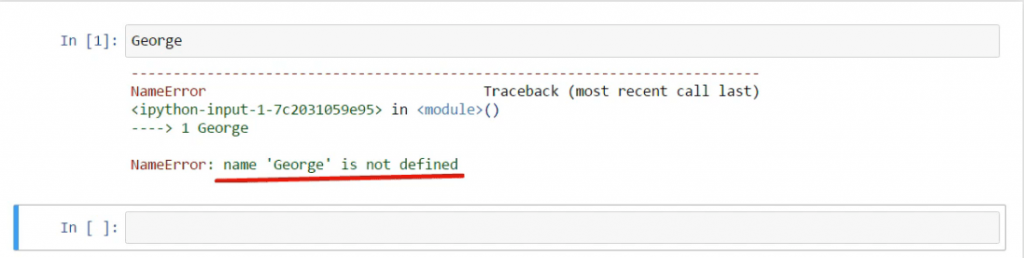If you are wondering why, it is because Python assumes George is the name of a variable to which we have assigned no value. Here’s the magic trick that will correct this mistake.

Typing quotation marks around the name George would do the job. We can put both single or double quotation marks to achieve this result.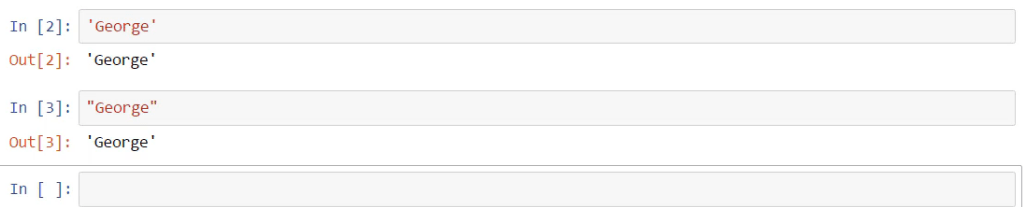The output values of these two inputs are the same. This is how Python displays text results if you don’t use the print command. Should you use print(), the output will be shown with no quotes – you’ll be able to see plain text.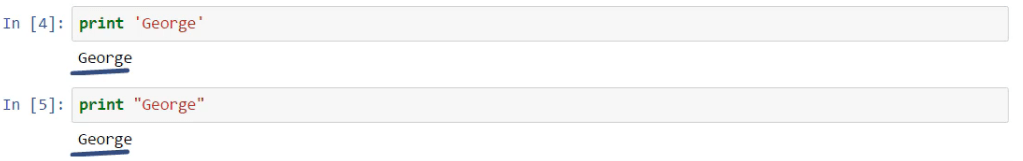If we assign “George” to a new variable, let’s say x4, we can obtain its output as we did with the integers and floats.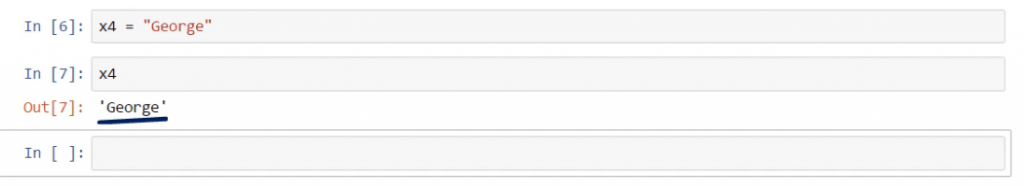All right, so that’s it. If the values you’d like to assign are not numerical, the quotes can come into play!

### How to Print Several Strings?

Let’s create the variable y which is supposed to represent the number of dollars you have in your pocket. In addition, we would like to ask the machine to print out a statement that says: “Y dollars”, where y is a number.

The proper way to combine the value of y and the string “Dollars” is to use a “+” sign, as shown below.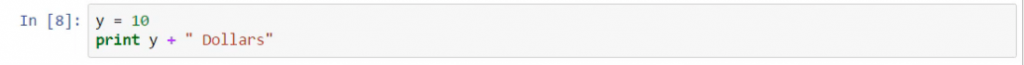And to check if we are missing something, we can execute the cell.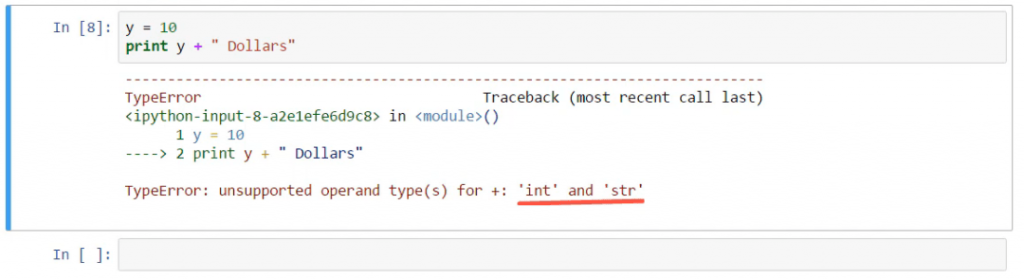Apparently, we did not respect the rules of coding in Python. We cannot put different types of variables in the same expression. Y is an integer, and “Dollars” is a string.

### How to Convert integers into strings?

We can convert y into a string. str() is the built-in function we need. Analogically to integers and floats, str() will convert our number into text, and that will unlock our result.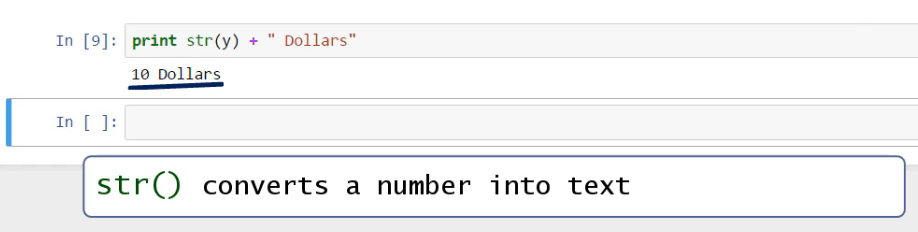To summarize, Python can automatically guess the type of data you are entering. It is within its capabilities to know for sure whether you have assigned an integer, a float, a Boolean, or a string. You need not declare the types of variables explicitly, as you must do in some other programming languages. Python always knows the type of variable and this is yet another reason why you should program in Python.

### Single vs Double Quotation

What will happen if we type something like “I’m fine”? We’ll need the apostrophe in the English syntax, not for the Pythonic one. Observe, if we execute the command like this, we will make a mistake.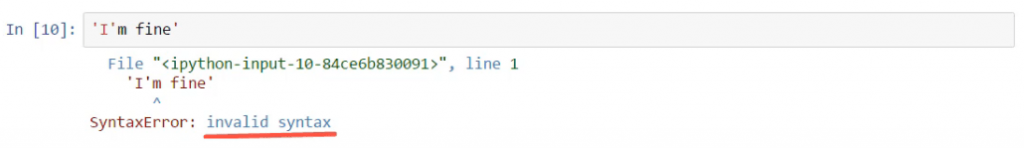To distinguish between the two symbols, let’s try putting the text within double quotes and leave the apostrophe, which technically coincides with the single quote.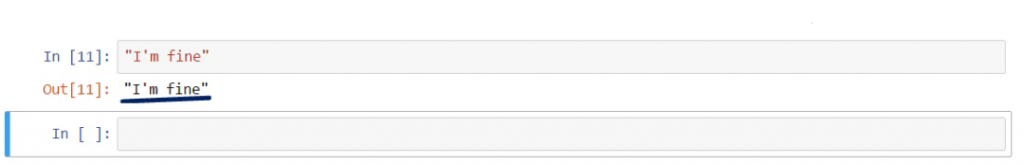An alternative way to do that would be to leave the quotes on the sides and place a backslash before the apostrophe within the phrase to obtain the same result. This backslash is called an escape character, as it changes the interpretation of the characters immediately after it.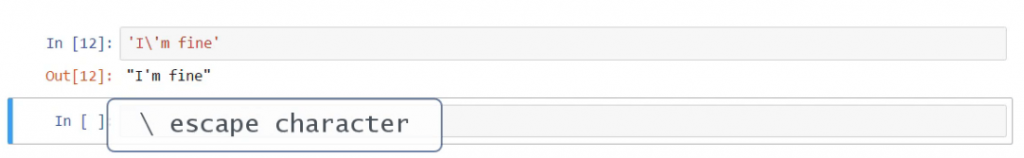And what if we wanted to state ‘press “Enter”’, where we put Enter within inverted commas? Same logic – the outer symbols must differ from the inner ones. For instance, we can put single quotes on the sides and obtain the desired result!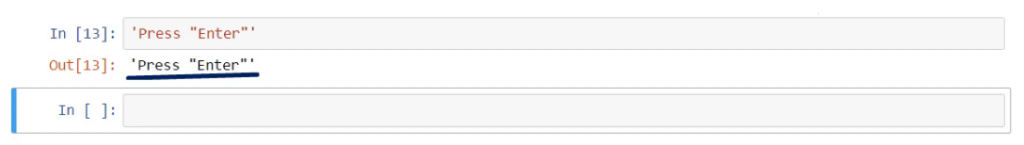## How to State Values?

Finally, let’s go through a few ways of stating values. Say, we wish to print the string “Red car” on the same line. If we write it like this – two words next to each other, separated by a blank space, we’ll see them attached.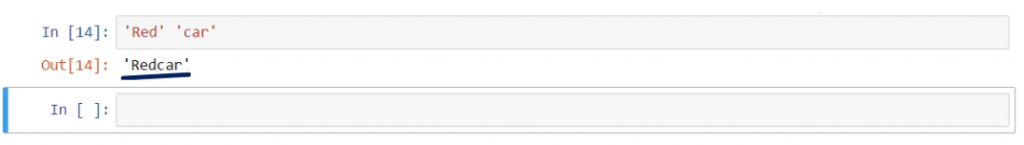One trick would be to put a blank space before the second apostrophe of the first word.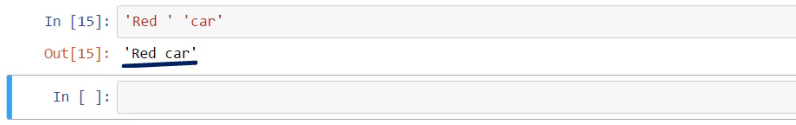Another technique would be to sort of “add” one of the strings to the other by typing in a plus sign between the two, just as we did with the “10-dollar” example before.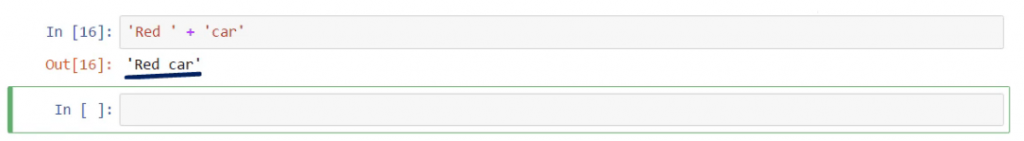As your intuition probably tells you, if we print this combination instead, we’ll obtain the same outcome, but it won’t have the quotes on the two sides.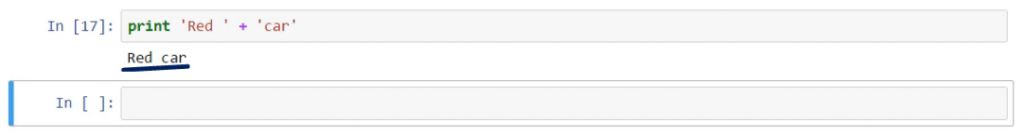How about we learn another trick we can do with the data types in Python? First, we can type “print ‘Red’", and then if we put a comma, which is called a trailing comma, Python will print the next word, ‘car’, on the same line, separating the two words with a blank space.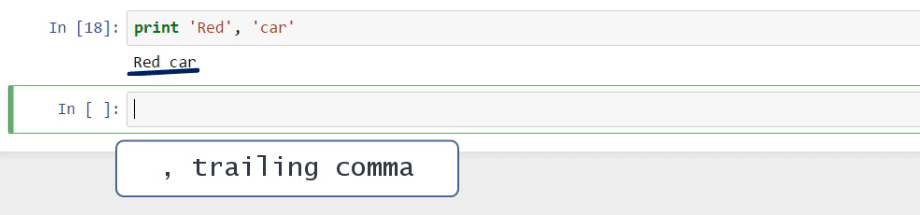Let’s prove this technique works for numeric values as well. Let’s print the number 3 next to the number 5.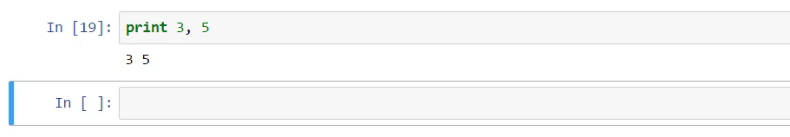What will happen if we don’t use the print() command and just list a few integers, floats, and strings separating them with commas? Python will execute the command as expected but will place the values within parentheses.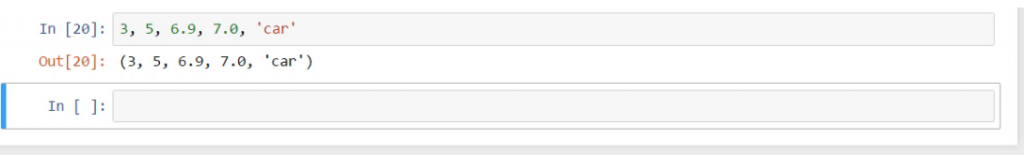### Understanding Data Types in Python

Now that you’ve mastered the basic data types in Python, you will be able to move on to the world of programming in Python! Provided that you want to improve your skills, you can go deeper into Python syntax.

***

If you’re enthusiastic about boosting your Python knowledge, check out our super practical tutorials!